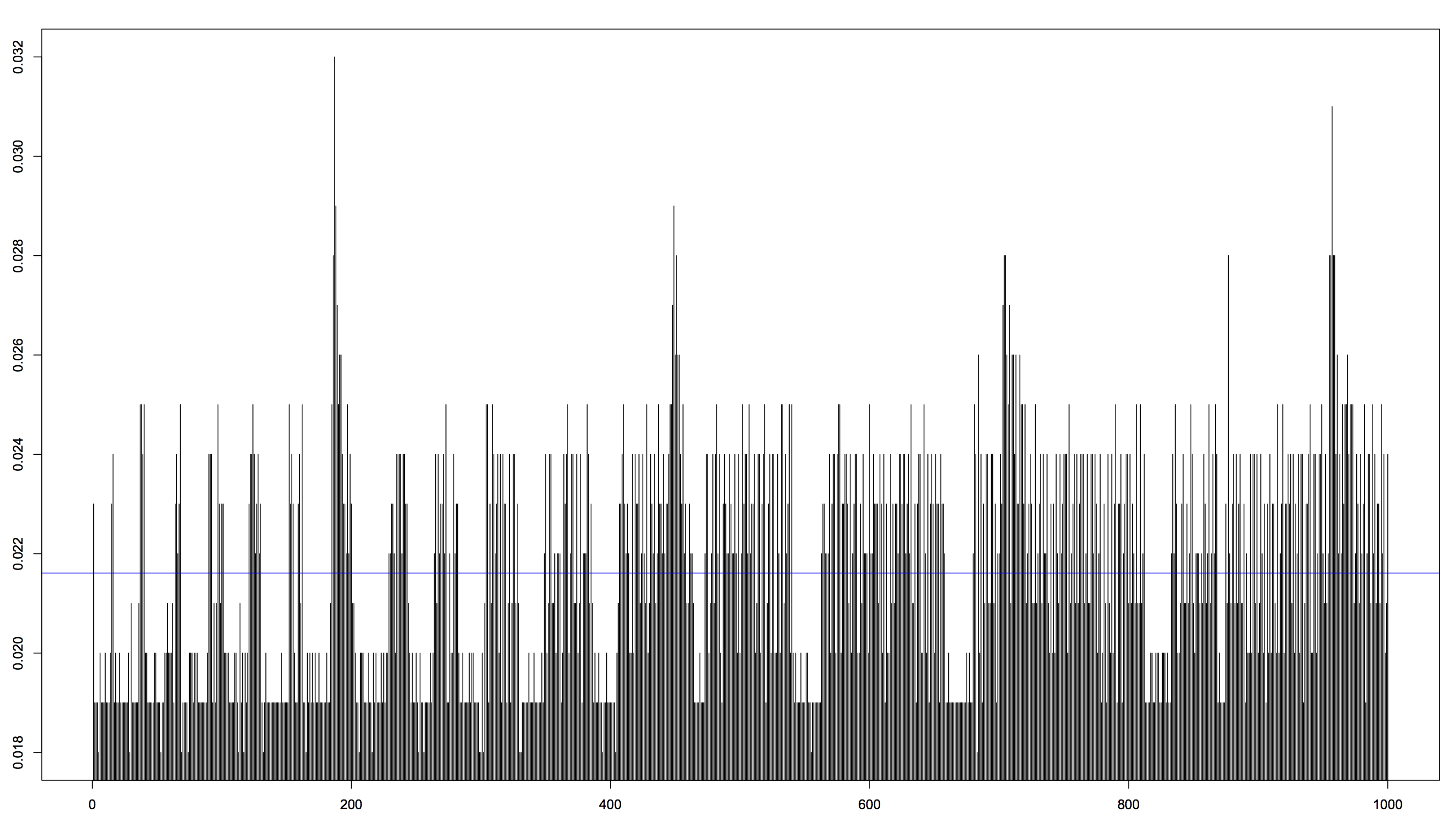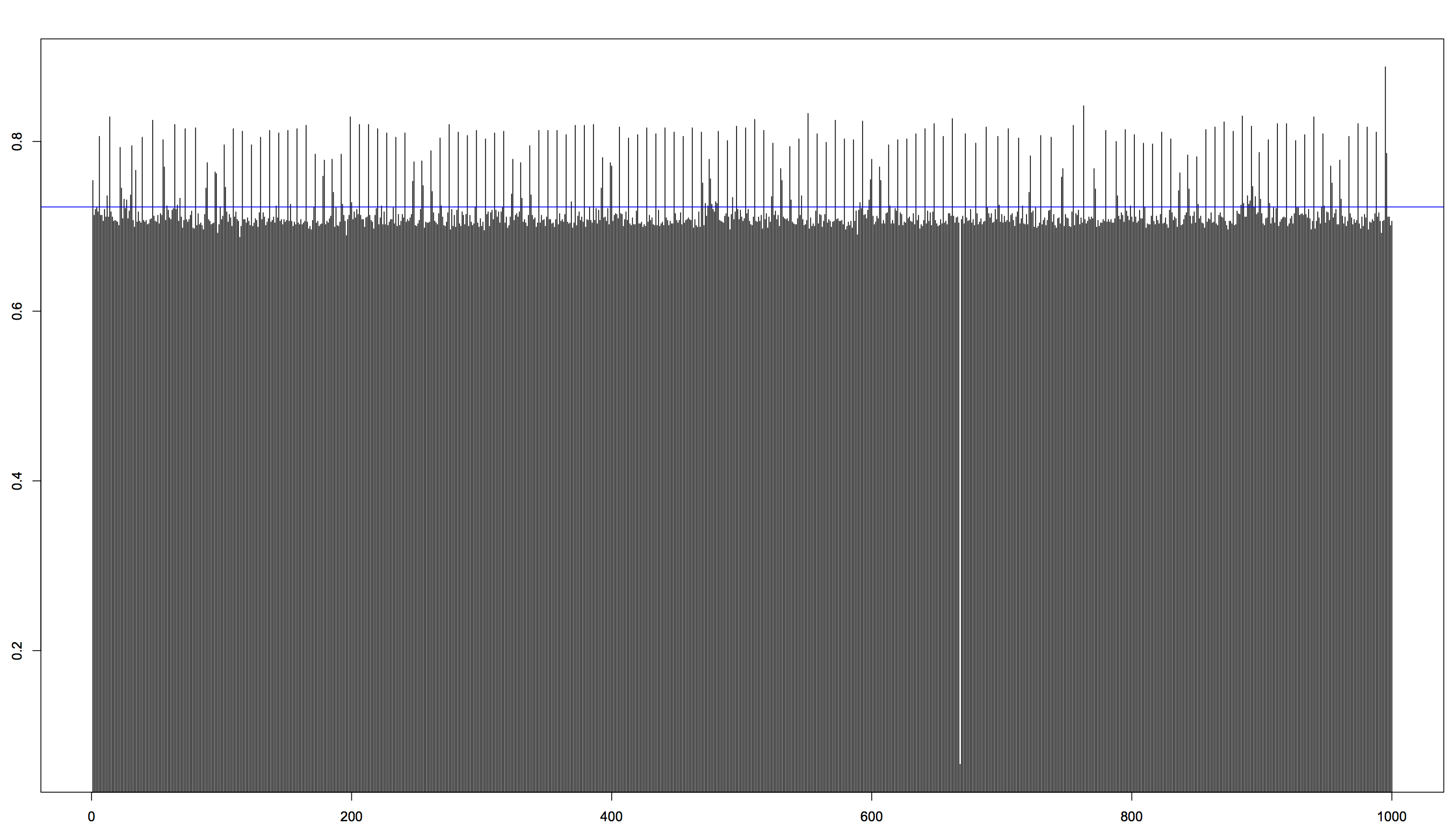Question: What do you do when you have two upcoming exams?

Answer: You get bored and start doing a performance tests between C and Python!

## The Metric

I decided to implement something quickly to find the 10001:st prime number (2 is the first prime). This implementation is not the fastest or the best performing one as far as I know, but since the object wasn’t to implement a fast algorithm I went for the easiest thing to implement. After all, we just want to compare how C and Python perform executing a similar algorithm.

NOTE: This is problem 7 in Project Euler so you might want to skip the code samples if you want to do the problems yourself.

## The Code

The source code is provided below, first in C and then in Python. I tried to match them as closely as possible.

``````#include<stdio.h>
#include<stdlib.h>
#include<math.h>

// Check if the number n is a prime.
int is_prime(int n)
{
int u = floor(sqrt(n));

for (int i = 2; i <= u; i++) {
if (n % i == 0) {
return 0;
}
}

return 1;
}

// Find the n:th prime number, counting 2 as the first one.
int prime_nbr(int n)
{
int c = 2; // The first prime
int i = 1; // We already got one prime (2)

while (i < n) {
c++;
if (is_prime(c)) {
i++;
}
}

return c;
}

int main(void)
{
int prime = prime_nbr(10001);
printf("%d\n", prime);

return 0;
}
``````
``````from math import sqrt,floor

def is_prime(n):
u = floor(sqrt(n))
i = 2
while i <= u:
if n % i == 0:
return False
i += 1

return True

def prime_nbr(n):
c = 2 # The first prime
i = 1 # We already got one prime (2)

while i < n:
c += 1
if is_prime(c):
i += 1

return c

prime = prime_nbr(10001)
print prime
``````

Now lets run them! But we can’t just run them once, then we might get skew the result for either language. Let’s run them 1000 times! (BTW, this took ages when running the Python version…)

``````for i in {1..1000}; do (time ./primec) 2>> log_c.txt; done
for i in {1..1000}; do (time python prime.py) 2>> log_py.txt; done
``````

The Unix program `time` came to mind as the easiest way to time the execution of both programs. To save all the execution times we redirect the output to two text files.

## The Result

After some magic in vim, we have two CSV files with the execution times.

``````0.023,0.019,0.019,0.019,0.018,0.020,... // Exec. times for C...

0.754,0.713,0.720,0.722,0.717,0.806,... // ...and Python
``````

Which can be read into R:

``````c <- scan(file='log_c.txt', what='list', sep=',')
p <- scan(file='log_py.txt', what='list', sep=',')
# For some reason, R insists on reading the values as strings
# so let's convert them
c <- as.numeric(c)
p <- as.numeric(p)
``````

To get some overview, let’s plot them in two histograms to see how it looks.Now, C is (obviously and expectedly) the faster one here. C’s mean value was 0.021611 s while Python’s was 0.722864 s, that’s approximately 33 times faster. Also, don’t be fooled by the fact that the execution time of C seems to jump around a lot more, it’s just different scales on the y-axis in the two plots. The standard deviation of C was 0.00229934 s while Python’s was 0.04084184 s, which means that Python actually jumped around a lot more than C when it comes to execution times.

## Summary

I would like to make it clear that I’m not doing this to bash Python in any way! I love Python and find it very pleasant to program in. I just did this for fun to get a break from the text book :)

Nevertheless, I did find it interesting that it was that big of a difference. I expect there are reasons why NASA used C to program Curiosity and not Python ^^